This browser does not support IFRAMEs - no Ads will appearBlueBonkers Home  >  Math WorkSheets  > Math homework exercises / math problem practice sheets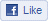BB on Facebook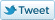BB on Twitter

## Math Practice WorkSheets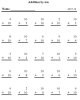Addition sheets grouped by the number of digits in the operation. Single digit(0-10), 2-Digit, 3-Digit, 4-Digit (with and without carry). Single digit has individual Practice sheets for each number such that all problems contain a fixed digit and a random alternate digit...top and bottom...great Practice!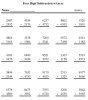### Subtraction Practice Sheets

Subtraction sheets grouped by the number of digits in the operation. Single digit(0-10), 2-Digit, 3-Digit, 4-Digit (with and without carry). Single digit has individual Practice sheets for each number such that all problems contain a fixed digit and a random alternate digit...top and bottom...great Practice!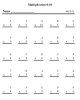### Multiplication Practice Sheets

Multiplication sheets grouped by the number of digits in the operation. Single digit(0-10), 2-Digit, 3-Digit, 4-Digit (with and without carry)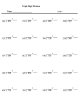### Division Practice Sheets

Division sheets grouped by the number of digits in the operation. Single digit(0-10), 2-Digit, 3-Digit, 4-Digit (with and without remainders)### Fractions Practice Sheets (all operations)

Our Fractions practice sheets contain: addition of fractions, subtraction, multiplication, division, and reduction of fractions sections !
Each of these sections includes several levels of complexity, such as: simple and mixed fractions along with examples of each with like and unlike denominators . Of course, all math practice sheets include separate answer sheets.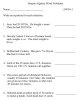### Simple Algebra Practice Sheets

Algebra sheets have multiple choice, true/false, and word problem selections all with a separate answer sheet.
These take three forms; Solve for a single Unknown variable, solve for Assigned variables, and (of course) Word problems .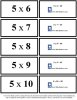### Flash Cards - Practice Sheets

We have created three sets of Flash Cards (multiplication and division) for single to low double digit operations (1-15);we also have geometric formula Flash Cards. Simply print on your local printer, fold in half and tape the open side. The problem appears on one side, while the problem and answer appears on the opposite side!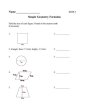### Geometry Practice Sheets

We have created practice sheets for simple to moderate level use of geometric formulas. (See above Flash Cards for formulas). Practice sheets include Area, Surface Area and and Volume practice sheets. Each practice sheet has a separate answer sheet.Activity Sheets
Activities for all ages
(K thru College)
Parent Friendly Activities:
• Coloring Page Sheets
• Cut-Outs
• Connect the Dots
• Scout/Camping Sheets

• Song Lyric Sheets
• Mother Goose Nursery
Rhymes and Stories

• Puzzle Sheets
Printable Puzzles for all ages and abilities:
• Sudoku Puzzles
• Number Puzzles
• Maze Puzzles
• Word Puzzles

• Math Sheets
Math Learning / Practice sheets by subject and ability level:
• Subtraction
• Multiplication
• Division
• Fractions
• Simple Algebra
• Geometry
• Flash Cards

• Online Coloring & Interactive Classic Games
Classic games that challenge and teach:
• Online Coloring
• Online Learning Games
• Online Fun Games

• Other Site Resources

• What's New
• Privacy / Terms
• Printing Tips

•